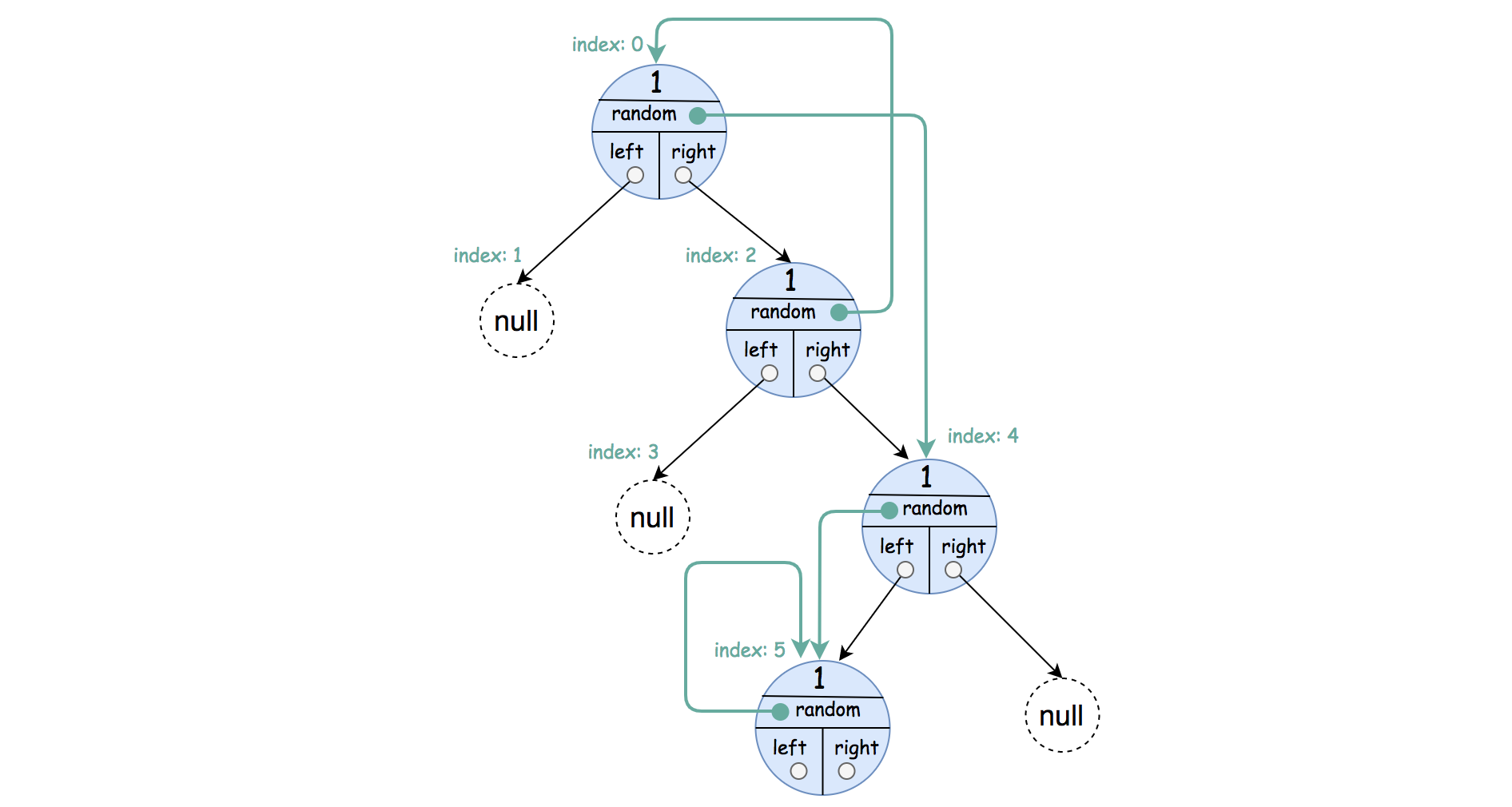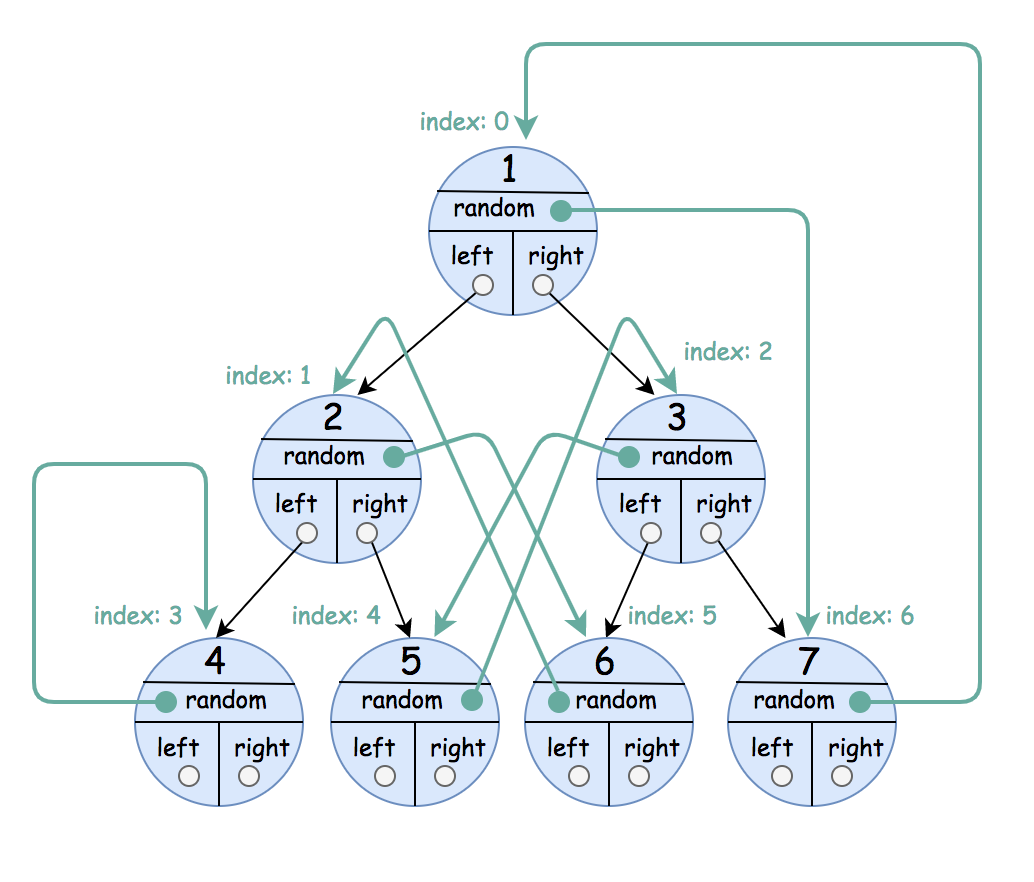Formatted question description: https://leetcode.ca/all/1484.html

# 1484. Clone Binary Tree With Random Pointer

Medium

## Description

A binary tree is given such that each node contains an additional random pointer which could point to any node in the tree or null.

Return a deep copy of the tree.

The tree is represented in the same input/output way as normal binary trees where each node is represented as a pair of [val, random_index] where:

• val: an integer representing Node.val
• random_index: the index of the node (in the input) where the random pointer points to, or null if it does not point to any node.

You will be given the tree in class Node and you should return the cloned tree in class NodeCopy.

Example 1:Input: root = [[1,null],null,[4,3],[7,0]]

Output: [[1,null],null,[4,3],[7,0]]

Explanation: The original binary tree is [1,null,4,7].

The random pointer of node one is null, so it is represented as [1, null].

The random pointer of node 4 is node 7, so it is represented as [4, 3] where 3 is the index of node 7 in the tree array.

The random pointer of node 7 is node 1, so it is represented as [7, 0] where 0 is the index of node 1 in the tree array

Example 2:Input: root = [[1,4],null,[1,0],null,[1,5],[1,5]]

Output: [[1,4],null,[1,0],null,[1,5],[1,5]]

Explanation: The random pointer of a node can be the node itself.

Example 3:Input: root = [[1,6],[2,5],[3,4],[4,3],[5,2],[6,1],[7,0]]

Output: [[1,6],[2,5],[3,4],[4,3],[5,2],[6,1],[7,0]]

Example 4:

Input: root = []

Output: []

Example 5:

Input: root = [[1,null],null,[2,null],null,[1,null]]

Output: [[1,null],null,[2,null],null,[1,null]]

Constraints:

• The number of nodes in the tree is in the range [0, 1000].
• Each node’s value is between [1, 10^6].

## Solution

Use a map to store each original node and the corresponding copied node. First, create the copied root and store the original root and the copied root into the map. Then create the copied tree recursively.

/**
* Definition for a binary tree node.
* public class Node {
*     int val;
*     Node left;
*     Node right;
*     Node random;
*     Node() {}
*     Node(int val) { this.val = val; }
*     Node(int val, Node left, Node right, Node random) {
*         this.val = val;
*         this.left = left;
*         this.right = right;
*         this.random = random;
*     }
* }
*/
class Solution {
Map<Node, NodeCopy> map = new HashMap<Node, NodeCopy>();

public NodeCopy copyRandomBinaryTree(Node root) {
if (root == null)
return null;
if (map.containsKey(root))
return map.get(root);
NodeCopy newRoot = new NodeCopy(root.val);
map.put(root, newRoot);
newRoot.left = copyRandomBinaryTree(root.left);
newRoot.right = copyRandomBinaryTree(root.right);
newRoot.random = copyRandomBinaryTree(root.random);
return newRoot;
}
}